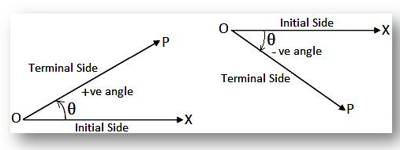Sign of Angles

An angle is made up of two rays with a common endpoint. This endpoint is the vertex of the angle. The angle symbol is a mathematical symbol that is placed ahead of character s, usually uppercase italic letters representing spatial points, to describe a geometric angle formed by the intersection of two lines, line segments, or rays. The symbol looks like a skewed, uppercase, sans-serif letter L (∠).Here, in the above figure, the angle is named as ∠ABC or ∠B.

Sign of Angles:

The definition of an angle is very useful in geometry.  In Trigonometry, we need a detailed definition of an angle.

Let a revolving line starting from OX, rotate about O in a plane and stop at position OP. Then it is said to trace out an angle XOP. OX is called initial side, OP is final or terminal side and O is the vertex of the angle. If the rotation is anticlockwise, the angle is positive. If the rotation is clockwise, the angle is negative.An angle is said to lie in a particular quadrant if the terminal side of an angle lies in the quadrant.

Information Source: